Courses

# Moment of Force about a Specific Axis Mechanical Engineering Notes | EduRev

## Mechanical Engineering : Moment of Force about a Specific Axis Mechanical Engineering Notes | EduRev

The document Moment of Force about a Specific Axis Mechanical Engineering Notes | EduRev is a part of the Mechanical Engineering Course Engineering Mechanics - Notes, Videos, MCQs & PPTs.
All you need of Mechanical Engineering at this link: Mechanical Engineering

Moment of a force about an axis

The moment of a force about an axis p is defined as the projection OB on p of the moment  (see Fig. 3.5).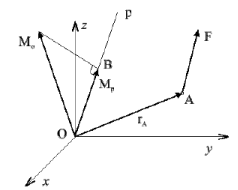Figure 3.5: Moment of the force F about the axis p.

Denoting λ the unit vector of p, the moment Mp of force F about an axis p can be expressed as scalar product (dot product)

Mp = λ . Mo      (3.22)

or as the mixed triple product of the unit vector λ , the position vector r, and the force F:

Mp = λ . ( r *F)     (3.23)

Using the determinant form for the mixed triple product, we have the magnitude of the moment

Moment of a force about an axis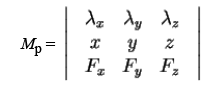(3.24)

λx,

where λyare direction cosines of axis p

λz

x, y, z are components of r

Fx,

Fy, Fz are components of F

Exercise 3.2.1

Sample problem A force F = 40 N is applied at a point M(xM;yM;zM) M(3;2;4) [m] (see Fig. 3.6). The line oF of action of the force F is described by direction angles (αFF;Cr ) (80o;60o; acute angle). An axis a which passes

through the origin O has direction angles (αa;βa;γa) ≡ (60o;100o; acute angle).

Determine:

• The moment Mo of the force F about O.
• The moments Mx, My, and Mz of the force F about axes x, y, and z, respectively.
• The moment Mof the force F about a axis.

Moment of a force about an axis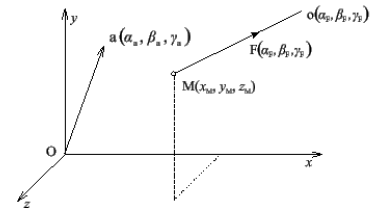Figure 3.6: Exercise 3.2.1. Moment of the force F about the axis a.

Notice: An acute angle is such an angle for which the condition 0 < α < 90o is valid.

Solution

We determine the angle Cr first. Since the expression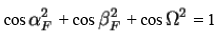is valid for any set of direction cosines, we have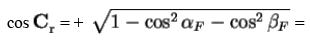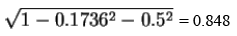According to the definition of the moment Mo of the force F, we may write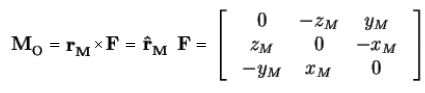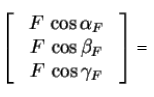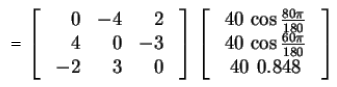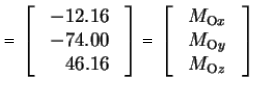Notice: Matlab works with radians and not with degrees.

The magnitude of Mo is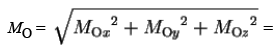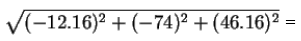88.06 Nm

The direction cosines of Mo are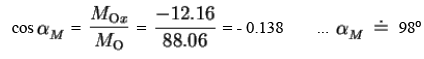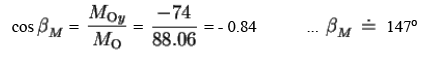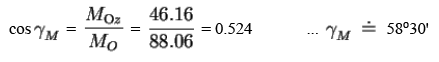As the components MOx, MOy, and MOz of Mo are equal to the moments Mx, My, and Mz of F about x, y, and z axes respectively, the following is valid:

Mx = MOx,    My = MOy,    Mz = MOz

According to the definition of the moment Ma of the force F about an axis a, we have

Ma = λT Mo

where the unit vector λ of a is

λ = [Cos αa, cosβa, cosγa]T

As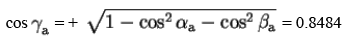we conclude that

Ma = [0.5, 0.1736, 0.8484]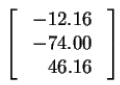= 45.92 Nm

Using MATLAB the solution of the problem is much more convenient. The program for the purpose is called smoment.m. It can be found in program package.

Exercise 3.2.2 Moment of a force A force F = 50 N is applied at a point M(8;4;4) [m] and its line of action is described by direction angles (60o; 60o; acute angle). Determine the moments Mx, My, and Mz of the force F about the axes x, y, and z respectively and the moment Ma about the axis a passing through the point A(0;2;2) and having direction angles (30o;0o; acute angle).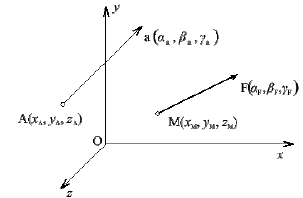Figure 3.7: Exercise 3.2.2. Moment of the force F about the axis a.

Solution

Mx = 41.4 Nm, My = -182.8 Nm, Mz = 100 Nm, Ma = -98.47 Nm

Exercise 3.2.3 Moment of a force A force F = 50 N is applied at a point M(2;3;1) [m] and its line of action is described by direction angles (60o; 60o; acute angle). The axis a passes through the origin O and lies in xy-plane having the angle  δ= 30o with y axis. Determine

• The moment Mo  of the force F about the origin O.
• The moments Mx, My, and Mz of the force F about the axes x, y, and z respectively.
• The moment Mof the force F about the axsis a.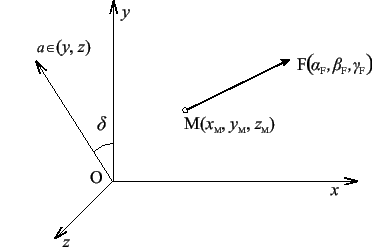Figure 3.8: Exercise 3.2.3. Moment of the force F about the axis a.

Solution

Mx = 81.05 Nm, My = -45.7 Nm, Mz = -25 N m, MO = 96.34 Nm, Ma = -52.07 Nm

Exercise 3.2.4 Moment of a force A force F = 40 N is applied at a point M(4;3;-2) [m] and its line of action is described by direction angles (30o; 90o; acute angle).
Determine the moment MA of the force F about the point A(-1;3;6) [m] and the moment Ma of the force F about the axis a passing through the point A and having direction angles (60o; 80o; acute angle).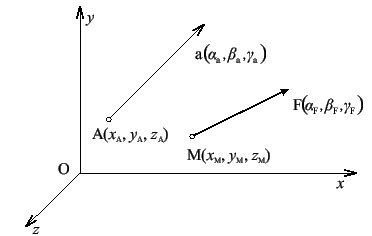Figure 3.9: Exercise 3.2.4. Moment of the force F about the axis a.

Solution

MAx = 0 Nm, MAy = -377.13 Nm, MAz = 0 Nm, Ma = -65.49 Nm

Exercise 3.2.5 Moment of a force A tube is welded to the vertical plate yz at the point A. The tube is loaded by a force F at the point D. The line of action of the force  has an angle β with y-axis and an angle γ with z-axis. Determine the moment of the force

F about the point A. Further determine the moment Mx about x-axis and the moment MBC about BC axis of the same force F . How many solutions does the task have? It is known that  F = 200 N; β = 60o; γ= 80o;a = 0.2 m;b = 0.4 m;c = 0.8 m.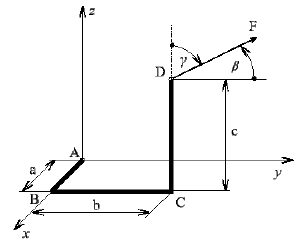Figure 3.10: Exercise 3.2.5. Moment of the force F.

Solution

MA = 152.2 Nm, Mx = - 66.1 Nm, MBC = 135.75 Nm

Exercise 3.2.6 Moment of a force Determine the moment of the force F = 500 N about the axis AC. The angle  α = 30o, A(5;0;0), C(0;12;0), E(0;0;20), AB = 6.5, BD = 10 (all dimensions in m).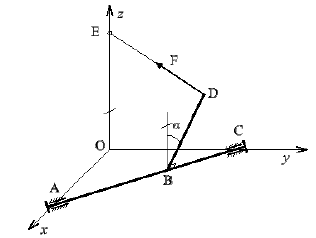Figure 3.11: Exercise 3.2.6. Moment of a force F.

Solution

MAC = 4510 Nm

Exercise 3.2.7 Tangent force A force T = 60 N acts at a tangent of a helix (see Fig.3.12). Find a generic expression for moments Mx, My, and Mz of the force T about x, y, and z axes respectively as a function of angle . Numerically compute the φ Numerically compute the magnitudes of these moments for φ =750o knowing that the radius of the helix is r =10 m and the pitch angle is  α =30o. Write a MATLAB program which can be used to calculate the above moments and use it for creating plots of moments values versus angle 0 ≤ φ ≤ 6π.Figure 3.12: Exercise 3.2.7. Tangent force.

Solution

Mx = 2222 Nm, My = -519.6 Nm, Mz = 3248 Nm

Offer running on EduRev: Apply code STAYHOME200 to get INR 200 off on our premium plan EduRev Infinity!

,

,

,

,

,

,

,

,

,

,

,

,

,

,

,

,

,

,

,

,

,

;